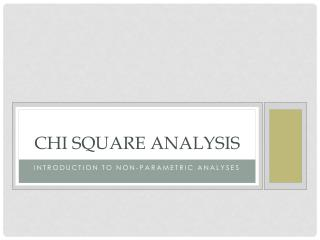# Chi Square Analysis - PowerPoint PPT PresentationDownload PresentationChi Square Analysis

Chi Square AnalysisDownload Presentation## Chi Square Analysis

- - - - - - - - - - - - - - - - - - - - - - - - - - - E N D - - - - - - - - - - - - - - - - - - - - - - - - - - -
##### Presentation Transcript

1. Chi Square Analysis Introduction to non-parametric analyses

2. Hypothesis Tests So far… • We’ve discussed • One-sample t-test • Dependent Sample t-tests • Independent Samples t-tests • One-Way Between Groups ANOVA • Factorial Between Groups ANOVA • One-Way Repeated Measures ANOVA • Correlation • Linear Regression • What do all of these tests have in common?

3. Parametric vs. Non-parametric • Parametric Tests – Statistical tests that involve assumptions about or estimations of population parameters. • (what we’ve been learning) • E.g., normal distribution, interval/ratio level measurement, homogeneity of variance • Nonparametric Tests • Also known as distribution-free tests • Statistical tests that do not rely on assumptions of distributions or parameter estimates • E.g., does not assume interval/ratio, no normality assumption • (what we’re going to be introducing today)

4. Some Non-parametric tests • Frequency Data • Chi-Square (2) Analysis • 2Goodness-of-Fit test (one variable) • 2Test of Independence (2 or more variables) • Non-normal Data (e.g., ordinal) • Mann-Whitney U (NP analogue of Independent Samples t-test) • Wilcoxon Signed Ranks Tests (NP analogue of Dependent Samples t-test) • Kruskal-Wallis One-Way Analysis of Variance (Between) • Friedman’s Rank Test for K correlated samples (Within)

5. Chi-Square • The2Goodness-of-Fit test • Used when we have distributions of frequencies across two or more categories on one variable. • Test determines how well a hypothesized distribution fits an obtained distribution. • The 2test of independence. • Used when we compare the distribution of frequencies across categories in two or more independent samples. • Used in a single sample when we want to know whether two categorical variables are related.

6. Chi-square Goodness of fit test • Quarter Tossing • Probability of Head? • Probability of Tails? • How can you tell if a Quarter is unfair when tossed? • Imagine a flipped a quarter 50 times, what would we expect?

7. Chi-square Goodness of fit test • Which of these scenarios seems probable with a “fair” coin?

8. Chi-square Goodness of fit test • We can compare it to our expectation about “fair” coins

9. Chi-square Goodness of fit test • We can test to see if our observed frequencies “Fit” our expectations • This is the2Goodness-of-Fit test • This converts the difference between the frequencies we observe and the frequencies we expect to a distribution with known probabilities

10. Chi-square Goodness of fit test • Hypothesis Test • H0: P(heads) = .5 • H1: P(heads) ≠ .5 • α = .05 • Type of test = 2 goodness-of-fit

11. Chi Square Distribution • DF = 2 – 1 = 1; See Chi-square table 2(1) = 3.841; If 2 observed is larger than 3.841, reject the null hypothesis

12. Chi-square Goodness of fit test • Do the test: For our coin example 7. Since 6.55 > 3.84, reject the null. There is evidence that the coin is not fair.

13. Chi-square test of independence • Used when we want to know if frequency responses of one categorical depend on another categorical variable (sounds like an interaction, right?)

14. Chi-square test of independence • We compare observed vs. expected frequencies as in the goodness-of-fit test but the expectant frequencies aren’t as easy to figure out because of the row and column totals.

15. Chi-square test of independence • Expectant frequencies for each cell is found by multiplying row and column totals then dividing by the grand total.

16. Chi-square test of independence • Example: Researchers stood on a corner and watched drivers come to a stop sign. They noted their gender and the type of stop they made.

17. Chi-square test of independence